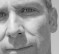Started by 4 years ago9 replieslatest reply 4 years ago85 views

Hi,

in this thread, @rbj mentioned that the MathJax implementation in this forum is erratic and I believe him. I had issued in the past and it is always frustrating.  I think most problems are due to conflicts between the wysiwyg editor and Mathjax.

If you find a minute or two, would you please use this thread here to reply and try to post an equation or two to possibly help me identify situations where Mathjax is not working as it should.

1- To post an equation, simply surround TeX code with a couple of dollar signs on each side:

$$E = mc^2$$


$$E = mc^2$$

2- If you want your equation to be displayed inline, use:

$$e^{i\pi}+1=0$$


$$e^{i\pi}+1=0$$

As I said, I suspect that most problems are caused by the editor that might screw up your equations after submitting/editing your post.  So if you are able to successfully post equations on your first try, please feel free to 'edit' your post and try to add equations to see if maybe post-editing might be causing problems.  Thanks a lot!

[ - ]$$R_{\mu\nu} - \frac{1}{ 2} R g_{\mu\nu} + \Lambda g_{\mu\nu} = \frac{8 \pi G }{c^4} T_{\mu\nu}$$

[ - ]First time it did not put out as an equation.  So I removed the space after the first $$and before the last$$ and then it worked.  I had an error in the first subscript, edited again, and it worked.  So for this case, the editing worked.

[ - ]HA!  And then I reloaded the page and the double dollar signs went from readable to functional in the reply.  So the mood of the editor seems to matter.

[ - ]I think that re-loading the page is always a good idea after submitting a reply, to make sure Mathjax runs on the new code.

In your case, you have 'and before the last' surrounded by two sets of dollar signs and Mathjax interprets the in-between as LaTex code.  Makes sense? Thanks Mike for your participation to this thread.

[ - ](I'm doing this from memory, don't sic Rudi Kalman on me):

$$P_n^- = F^T P_{n-1}^+ F + Q$$

[ - ]This isn't rendering (at least not the first time):

$$\dot x = A x + B (k \hat x)$$

[ - ]Let me try.

$$\dot x = A x + B (k \hat x)$$

[ - ]I was able to make the mathjax code work by editing the html (<>).

<p>$$</p> <p>\dot x = A x + B (k \hat x) </p> <p>$$
</p>


After removing the html in between the dollar signs:

$$\dot x = A x + B (k \hat x)$$
$$\dot x = A x + B (k \hat x)$$
[ - ]I thought the linefeeds might be messing things up.  Hmm.  Should be $$\dot x = A x + B\,(k\,\hat x)$$